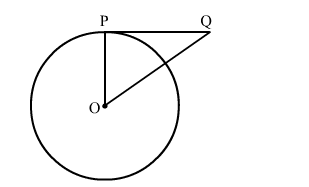# PQ is a tangent to a circle with centre O at the point P.`
Question:

PQ is a tangent to a circle with centre O at the point P. If △OPQ is an isosceles triangle, then ∠OQP is equal to

(a) 30
(b) 45
(c) 60
(d) 90

Solution:We know that the radius and tangent are perperpendular at their point of contact
Now, In isoceles right triangle POQ
∠POQ + ∠OPQ + ∠OQP = 180            [Angle sum property of a triangle]
⇒  2∠OQP + 90 = 180
⇒ ∠OQP = 45
Hence, the correct answer is option (b).